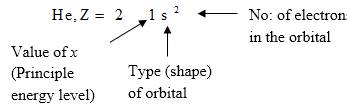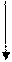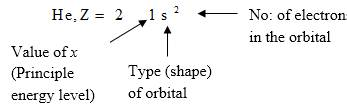Chapter 12, Problem 44CR### Introductory Chemistry: A Foundati...

9th Edition
Steven S. Zumdahl + 1 other
ISBN: 9781337399425

#### Solutions

Chapter
Section### Introductory Chemistry: A Foundati...

9th Edition
Steven S. Zumdahl + 1 other
ISBN: 9781337399425
Textbook Problem
1 views

# Write the electron configuration for the following atoms, using the appropriate noble gas to abbreviate the configuration of the core electrons.msp;  a .  Sr ,  Z  =  38                                                 d .  K ,  Z  =  19 b .  AL ,  Z  =  13                                             e .  S ,  Z  =  l6 c .  Cl ,  Z  =  l7                                                       f .  As ,  Z  =  33

Interpretation Introduction

(a)

Interpretation:

Determine the electron configuration of atoms using the appropriate noble gas configuration of the core electrons.

Concept Introduction:

Electron configuration describes the positions of electrons of an atom. These positions were explained by several models developed by different scientists. Principal energy level, Type (shape) of orbital and No: of electrons in the orbital are respectively represented by an atom’s electron configuration.Explanation

Order in which orbitals fill to produce the atoms in periodic table as follows:

1s22s22p63s23p64s23d104p65s24d105p66s24f145d106p67s25f146d107p6

There are only one s orbital, three no: of p orbitals, five no: of d orbitals and seven no: of f orbitals can be present and every one of above mentioned orbitals include, maximum two no: of electrons which are opposite in spin.

Noble gas configurations are as follows:

Period No

He, Z= 2 ;  1s2

Ne, Z= 10 ; 1s22s22p6

Ar, Z= 18 ; 1s22s22p63s23p6

Kr, Z= 36 ; 1s22s22p63s23p

Interpretation Introduction

(b)

Interpretation:

Determine the electron configuration of atoms using the appropriate noble gas configuration of the core electrons.

Concept Introduction:

Electron configuration describes the positions of electrons of an atom. These positions were explained by several models developed by different scientists. Principal energy level, Type (shape) of orbital and No: of electrons in the orbital are respectively represented by an atom’s electron configuration.Interpretation Introduction

(c)

Interpretation:

Determine the electron configuration of atoms using the appropriate noble gas configuration of the core electrons.

Concept Introduction:

Electron configuration describes the positions of electrons of an atom. These positions were explained by several models developed by different scientists. Principal energy level, Type (shape) of orbital and No: of electrons in the orbital are respectively represented by an atom’s electron configuration.Interpretation Introduction

(d)

Interpretation:

Determine the electron configuration of atoms using the appropriate noble gas configuration of the core electrons.

Concept Introduction:

Electron configuration describes the positions of electrons of an atom. These positions were explained by several models developed by different scientists. Principal energy level, Type (shape) of orbital and No: of electrons in the orbital are respectively represented by an atom’s electron configuration.Interpretation Introduction

(e)

Interpretation:

Determine the electron configuration of atoms using the appropriate noble gas configuration of the core electrons.

Concept Introduction:

Electron configuration describes the positions of electrons of an atom. These positions were explained by several models developed by different scientists. Principal energy level, Type (shape) of orbital and No: of electrons in the orbital are respectively represented by an atom’s electron configuration.Interpretation Introduction

(f)

Interpretation:

Determine the electron configuration of atoms using the appropriate noble gas configuration of the core electrons.

Concept Introduction:

Electron configuration describes the positions of electrons of an atom. These positions were explained by several models developed by different scientists. Principal energy level, Type (shape) of orbital and No: of electrons in the orbital are respectively represented by an atom’s electron configuration.### Still sussing out bartleby?

Check out a sample textbook solution.

See a sample solution

#### The Solution to Your Study Problems

Bartleby provides explanations to thousands of textbook problems written by our experts, many with advanced degrees!

Get Started